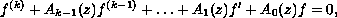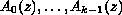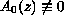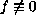Electron. J. Diff. Eqns., Vol. 2003(2003), No. 17, pp. 1-12.

### Order and hyper-order of entire solutions of linear differential equations with entire coefficients Benharrat Belaidi & Karima Hamani

Abstract:
In this paper, we investigate the growth of solutions of the differential equationwhereare entire functions with. We will show that if the coefficients satisfy certain growth conditions, then every finite order solution of the equation will satisfy certain other growth conditions. We will also find conditions on the coefficients so that every solutionwill have infinite order and we estimate in one case the lower bounds of the hyper-order.

Submitted November 27, 2002. Published February 20, 2003.
Math Subject Classifications: 30D35, 34M10, 34C11.
Key Words: Linear differential equations, growth of entire functions, hyper-order.

Show me the PDF file (217K), TEX file, and other files for this article.Benharrat Belaidi Department of Mathematics Laboratory of Pure and Applied Mathematics University of Mostaganem, B. P 227 Mostaganem Algeria e-mail: belaidi@univ-mosta.dz, belaidi.benharrat@caramail.com Karima Hamani Department of Mathematics Laboratory of Pure and Applied Mathematics University of Mostaganem, B. P 227 Mostaganem Algeria